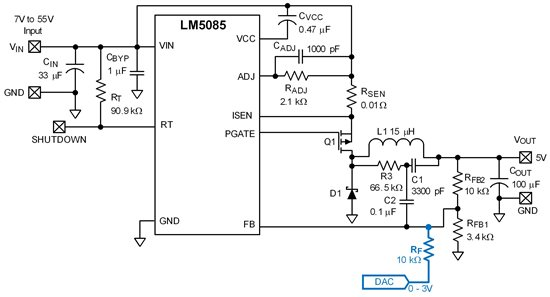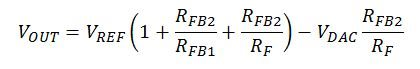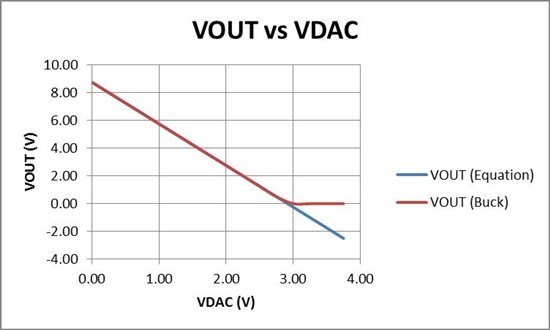# How to dynamically control the output voltage with a COT regulator

Constant-On-Time (COT) regulators provide a simple, cost-effective way of implementing step-down buck regulators with nearly fixed frequency. COT regulators do not require loop compensation and provide excellent transient performance with minimum design effort. Non-synchronous operation results in reduced switching frequency at very light load resulting in higher efficiency than a comparable fixed frequency converter.

In many applications you must control the output voltage of a converter dynamically through an external control signal.  Some low voltage converters and controllers have a dedicated control or track input to achieve this task, but most wide VIN converters don’t. In this blog, I demonstrate a universal method of dynamically controlling the output voltage of a DC/DC converter through the feedback pin. COT converters are well-suited for variable output voltage applications since the change in output voltage does not affect the closed loop stability of the converter.

Buck Application Circuit with Dynamic Output Voltage ControlCurrent Injection at Feedback Node

The output voltage relationship with the applied control voltage is given byor in an alternate formwhich shows that the maximum VOUT is achieved when VDAC is the minimum. If the minimum VOUT from the above equation is negative, it is clipped to VOUT=0V – the minimum output voltage of a buck converter – when operating from a unipolar supply.

Control Voltage (VDAC) vs Output Voltage (VOUT)
RFB2=10 kΩ, RFB1=3.4 kΩ, RF=10 kΩResources:

• The plot corresponds to RF = 3.4k, not 10k, so VOUT = 8.6V - 2.94*VDAC.

• I don't see how the numbers add up. Am I doing something wrong?

VREF(1+RFB2/RFB1+RFB2/RF) - VDAC*RFB2/RF

VREF(1+10k/3.4k+10k/10k)-VDAC*10k/10k

VREF*4.94-VDAC

LM5085 VREF is 1.25V

1.25V*4.94 -VDAC

=6.175V-VDAC

This means the DAC can set the output voltage between 3.175V and 6.175V. I don't see how the transfer function in the graph can be accurate. What am I doing wrong?